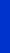School of Mathematical and Physical Sciences, Department of Mathematics Dimitrios E. TzanetisNational Technical University of AthensEmeritus Professor
 E-mail:    dtzan@math.ntua.gr                                                     dtzan@central.ntua.gr
 Dimitrios  E. Tzanetis
 Phone: + 30 210 772 1756Mail Address: Department of Mathematics, School of Mathematical and Physical Sciences, National Technical University of Athens, Zografos Campus,  GR-157 80,  Athens,  GreeceHome
 Dimitrios Tzanetis is in the Department of Mathematics, of the School of Mathematical and Physical Sciences (N.T.U.A.), where he has worked since 1976. He graduated from the School of Mathematics, Aristotle University of Thessaloniki in 1973 and completed his Ph.D. in Mathematics at Heriot Watt University, Edinburgh, in 1986 under the supervision of Prof. Andrew Lacey. His research interests focus on the following areas: · Partial Differential Equations, (Parabolic and hyperbolic type initial-boundary value problems, Elliptic boundary value problems, Local and non-local problems modelling Ohmic Heating ) · Ordinary Differential Equations (Population dynamics) · Mathematical Modelling · Asymptotic Analysis